# Lecture 5 Collisionless Boltzmann Equation

## Collisionless dynamics

A typical galaxy has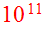stars but is onlycrossing times old, so the cumulative effects of encounters between stars are not significant. This justifies the next step, which is to idealize a galaxy as a continuous mass distribution. In this limit, each star moves in the smooth gravitational field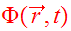of the galaxy. Thus instead of thinking about motion in a phase space ofdimensions, we can think about motion in a phase space of just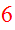dimensions. This is a very important simplification!

## Distribution function

Rather than keeping track of individual stars, a galaxy may be described by the one-body distribution function; letbe the mass of stars in the phase-space volume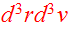at (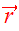,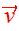) and time. This provides a complete description if stars are uncorrelated, as assumed above.

## Collisionless Boltzmann equation

The motion of matter in phase space is governed by the phase space flow,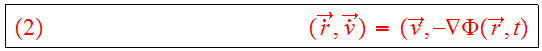How does this affect the total amount of mass in the phase space volume? The rate of change of the mass is just the inflow minus the outflow; that is, the flow obeys a continuity equation in 6 dimensions:where the derivatives with respect to, andare understood to be partial derivatives. Using the expression for the phase-flow yields the collisionless Boltzmann equation:The collisionless Boltzmann equation or CBE describes the evolution of the distribution function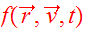. It serves as the fundamental equation of galactic dynamics.

## Fluid continuity equation

(See explanation with added class note here)

## Gravity

The gravitational field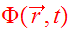is given self-consistently by Poisson's equation,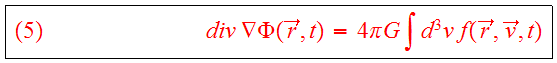Eqs. (4,5) may be viewed as a pair of coupled PDEs which together completely describe the evolution of a galaxy.

## Conservation of phase space density

Letbe the orbit of a star. What is the rate of change ofalong the star's orbit? The answer is zero,where the first equality is just the definition of the convective derivative in phase-space, the second equality follows on substituting the phase-flow (Eq. 2), and the last equality follows from the CBE (Eq. 5). Thus, phase-space density is conserved along every orbit.

This fundamental and completely general result shows that the CBE has a much greater level of symmetry than the N-body equations of motion; whereas the latter conserves a fairly small set of parameters, the CBE conserves f(r,v,t) along an infinite number of stellar orbits. We can take advantage of this infinite array of conservation laws to obtain some important results even when we can't explicitly solve the CBE.